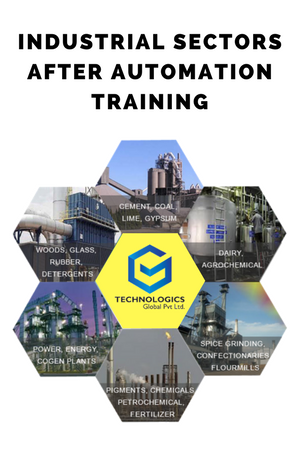### Post Graduate Diploma in AI & ML Training

Introduction to Python/R

• Why Python/R Programming.
• Data Types And Operators.
• Control Flow, Functions & Scripting.
• Numpy, Pandas, And Matplotlib.
• Anaconda, Jupyter Notebooks, Numpy Basics, Pandas Basics, Matplotlib Basics.
• Linear Algebra Essentials:
• Introduction Vectors, Linear Combination, Linear Transformation And Matrices, Linear Algebra In Neural Networks
• Lab: Vectors Lab, Linear Combination Lab, Linear Mapping Lab
• Neural Networks: Introduction To Neural Networks
• Training Neural Networks
• Deep Learning With Tensorflow
• Project: Image Classifier With Tensorflow
COURSE DETAILS

• Instructor(s) : Corporate Trainer
• Fast-Track : 1 months
• Normal-Track : 1.5 – 2 months
• Course Name : PGAIML

CONTACT DETAILS

• Batch Starts : Call @ +91 9738171920
• Level : Intermediate
• Timings (NT) : 3 Hours/Day (Normal Track)
• Timings (FT) : 6 Hours/Day (Fast Track)# Training BrandsWhy AI & ML ?

This Artificial Intelligence course You will master TensorFlow, Machine Learning, and other AI concepts, plus the programming languages needed to design intelligent agents, deep learning algorithms & advanced artificial neural networks that use predictive analytics to solve real-time decision-making problems.
Why To Choose us ??

Training Brands
Training Highlights
Hands-on Practical Training at State Of Art Lab
Find More About Technologics
Training Specialization
Students Reviews